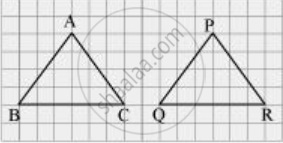Share

# In a Squared Sheet, Draw Two Triangles of Equal Areas Such that the Triangles Are Congruent. What Can You Say About Their Perimeters? - CBSE Class 7 - Mathematics

ConceptCongruence of Triangles

#### Question

In a squared sheet, draw two triangles of equal areas such that

The triangles are congruent.

What can you say about their perimeters?

#### SolutionHere, ΔABC and ΔPQR have the same area and are congruent to each other also. Also, the perimeter of both the triangles will be the same.

Is there an error in this question or solution?

#### APPEARS IN

NCERT Solution for Mathematics for Class 7 (2018 to Current)
Chapter 7: Congruence of Triangles
Ex. 7.20 | Q: 7.1 | Page no. 150
Solution In a Squared Sheet, Draw Two Triangles of Equal Areas Such that the Triangles Are Congruent. What Can You Say About Their Perimeters? Concept: Congruence of Triangles.
S